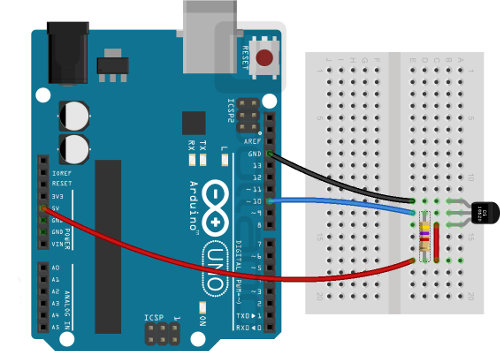# Arduino in combination with DS18B20 temperature sensor

This guide shows how to operate the temperature sensor via the Arduino board.

#### Connection

At the beginning the library should be added to Arduino environment (Sketch -> Include Library -> Add .ZIP Library...). Next we connect sensor pins according to the table below:

Arduino pins Pin Sensor
GND GND
Pin 10 DQ
5 V Vdd

 In addition, the sensor requires a 4.7 kΩ pull-up resistor between DQ and 5 V power supply - this does not apply to modules that already have this resistor built in.Wiring diagram of the temperature sensor.

#### Operation

In order to obtain the temperature from the sensor we will use a sample program DS18x20_Temperature from the attached library (File -> Examples -> OneWire -> DS18x20_Temperature).

```
// OneWire DS18S20, DS18B20, DS1822 Temperature Example
//
// http://www.pjrc.com/teensy/td_libs_OneWire.html
//
// The DallasTemperature library can do all this work for you!
// " http://milesburton.com/Dallas_Temperature_Control_Library

OneWire ds(10); // on pin 10 (a 4.7K resistor is necessary)

void setup(void) {
Serial.begin(9600);
}

void loop(void) {
I was and;
byte present = 0;
byte type_s;
byte date;
float celsius, fahrenheit;

Serial.println();
ds.reset_search();
delay(250);
return;
}

Serial.print("ROM =");
for( i = 0; i < 8; i++) {
Serial.write('');
}

Serial.println("CRC is not valid!");
return;
}
Serial.println();

// The first ROM byte indicates which chip
case 0x10:
Serial.println(" Chip = DS18S20"); // or old DS1820
type_s = 1;
break;
case 0x28:
Serial.println(" Chip = DS18B20");
type_s = 0;
break;
case 0x22:
Serial.println(" Chip = DS1822");
type_s = 0;
break;
default:
Serial.println("Device is not a DS18x20 family device.");
return;
}

reset();
ds.write(0x44, 1); // start conversion, with parasite power on at the end

delay(1000); // maybe 750ms is enough, maybe not
// we might do a ds depower here, but the reset will take care of it.

present = ds.reset();

Serial.print(" Data = ");
Serial.print(present, HEX);
Serial.print(" ");
for ( i = 0; i < 9; i++) { // we need 9 bytes
Serial.print(data[i], HEX);
Serial.print(" ");
}
Serial.print(" CRC=");
Serial.print(OneWire::crc8(date, 8), HEX);
Serial.println();

// " Convert the data to actual temperature
// because the result is a 16 bit signed integer, it should
// be stored to an "int16_t" type, which is always 16 bits
// even when compiled on a 32 bit processor.
int16_t raw = (date << 8) | date;
if (type_s) {
raw = raw << 3; // 9 bit resolution default
if (date == 0x10) {
// count remain gives full 12 bit resolution
raw = (raw & 0xFFF0) + 12 - date;
}
} else {
byte cfg = (date & 0x60);
// " at lower res, the low bits are undefined, so let's zero them
if (cfg == 0x00) raw = raw & ~7; // 9 bit resolution, 93.75 ms
else if (cfg == 0x20) raw = raw & ~3; // 10 bit res, 187.5 ms
else if (cfg == 0x40) raw = raw & ~1; // 11 bit res, 375 ms
//// default is 12 bit resolution, 750 ms conversion time
}
celsius = (float)raw / 16.0;
fahrenheit = celsius * 1.8 + 32.0;
Serial.print(" Temperature = ");
Serial.print(celsius);
Serial.print(" Celsius, ");
Serial.print(fahrenheit);
Serial.println(" Fahrenheit");
}
```

The effects of the program can be seen on the screen below.Serial screenshot of the monitor.

## Botland.store - shop for makers!

Otrzymaj rabat 5% na pierwsze zakupy Join to newsletter